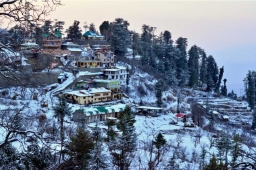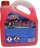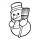# Cooler place

The temperature recorded in Simla is -4°C and in Kufri is -6°C on the same day which place is cooler on the day?

Result

p = (Correct answer is: Kurfi)### Step-by-step explanation:Did you find an error or inaccuracy? Feel free to write us. Thank you!## Related math problems and questions:

• The temperature 11The temperature on Sunday was 4°C. The temperature dropped 8°C on Monday and twice as much on Tuesday. What is the temperature on Tuesday?
• The temperature 10The temperature in a freezer is -15°C, and it increases by 3°C.
• OpheliaOphelia recorded the temperature of a cold store every two hours. 1. At 6 am, it was -4°C, and at 8 am, it was -1°C. By how much did the temperature rise? 2. The temperature went up by 5°C in the next two hours. .What was the temperature at 10 am?
• Temperature difference 2The temperature in London on new year’s day is -2 degree Celsius. The temperature in Moscow on the same day is -14 degree Celsius, what is the temperature difference between the two cities?
• If the 2If the temperature is 86 degrees in Fahrenheit, what is the same temperature in Celsius?
• WeatherWeather. At 5:00 am, the temperature was 28°C, and at noontime, it rose by 9°C. By 4:00 pm, it dropped 3°C, and by nighttime, it was 4°C less. What is the room temperature this time?
• The lowestThe lowest temperature on Thursday was 20°C. The lowest temperature on Saturday was 12°C. What was the difference between the lowest temperatures?
• LaboratoryMang Elio went to his laboratory at 7:00am And recorded that the temperature was 11.07°C. At lunchtime, the temperature rose by 27.9°C but dropped by 8.05°C at 5:00 PM. What was the temperature at 5:00 PM?
• Temperature 13Work out the temperature after each change. The temperature starts at 6°C and falls by 13°C. The temperature starts at 2°C and falls by 8°C
• The temperature 8The temperature on Monday was –6°C. On Tuesday, the temperature was 3 degrees lower. Write down the temperature on Tuesday.
• ThermometerThe thermometer showed -12 degrees Celsius in the morning then the temperature rises by 4 degrees and later again increased by 2 degrees at the evening has fallen by 5 degrees and then falls 3 degrees. What end temperature does the thermometer show?
• 8 degrees8 degrees Celsius it cools down by 9 degrees Celsius. What is the resultant temperature?
• Temperature differenceLibya's highest temperature was recorded at 58 degrees Celsius, and the lowest was recorded at -88 degrees Celsius. What is the temperature difference?
• Change in temperatureStarting temperature is 21°C, the highest temperature is 32°C. What is the change in temperature?
• Celsius 25 degrees Celsius at midday dropped 12°Celsius degree by evening. What is the temperature?
• ThermometerThe thermometer showed -3°C in the morning. Then the temperature was increased by 1°C again increased by 1°C and then decreased by 1°C and then decreased by 4°C. Which terminal temperature thermometer shows?
• Temperature increaseIf the temperature at 9:am is 50 degrees. What is the temperature at 5:00pm if the temperature increases 4 degrees Fahrenheit each hour?